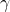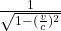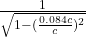## How much work must be done on a particle with a mass of mm to accelerate it from rest to a speed of 0.084 cc ? (Express the answer in terms

Question

How much work must be done on a particle with a mass of mm to accelerate it from rest to a speed of 0.084 cc ? (Express the answer in terms of mc2mc2.)

in progress 0
2 weeks 2021-09-05T15:00:36+00:00 1 Answers 0 views 0

Work = 0.68 mc²

Explanation:

given data

speed = 0.084 cc

solution

as kinetic energy of mass particle is

KE = mc²(– 1 )  …………1

hereis lorentz constant===  1.68

as here particle start from rent so initial KE is 0

and final KE = mc²(1.68 – 1 )

final KE = 0.68mc²

so here work done to increase speed of particle

W = final KE – initial KE

Work = 0.68 mc²